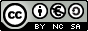Maths Workshop INDEX Introduction Aims Let's play Nim Learning to count Changing base How can we win at Nim? Another game of Nim A different game
NUMBER SYSTEMS
INTRODUCTION

Counting was one of the first mathematical activities that primitive man had to learn how to do. Expressing a quantity with symbols meant a great step forward as they were the first number systems. However, they were awkward to work with. The decimal system and place-value notation, which we use nowadays, was thought up in India (VI - IX Century) and the Arabs introduced it to the Western world (X century) where it started to be used in the XIII century.

We use the decimal number system as we have 10 fingers (the hand was the first calculator in history). The main advantage of this system is that it uses place-value notation (or positional notation) as the value of each figure depends on its position. However, there are other number systems too. For example, the theoretical mechanism which is used to make computers work is based on the binary number system.

This unit is based on a game called Nim. The strategy used to win the game is to make certain calculations in base 2. The game is used as a way of introducing some initial concepts about number systems.

Many scientific calculators used these days (including the one which comes with the operating system in your computer) can be used to work with other number systems: in binary (base 2), in octal (base 8) and in hexadecimal (base 16). You could investigate them when you have finished this unit.

Visit the following Internet web pages to find out more information (in Spanish) about number systems:

http://thales.cica.es/rd/Recursos/rd97/Otros/SISTNUM.html

http://geocities.com/aritmeticarecreativa/cap04.html

http://www.sectormatematica.cl/historia.htm

For more games like the Nim game go to http://www.cut-the-Knot.com/ctk/May2001.html

AIMS
• To investigate by playing.
• To understand the concept of a base in a number system.
• To count using different number systems.
• To understand the principle of the relative value
• To express any number written in base 10 in another base.
• To express a number written in any other base in base 10.
• To express a number written in base m in base n.
• To use base 2 as a strategy to win at Nim.

 Juan Simón SantamaríaSpanish Ministry of Education. Year 2002